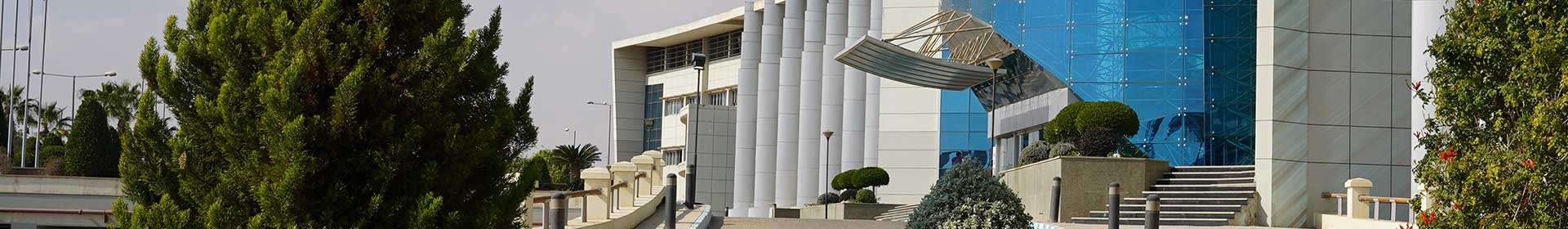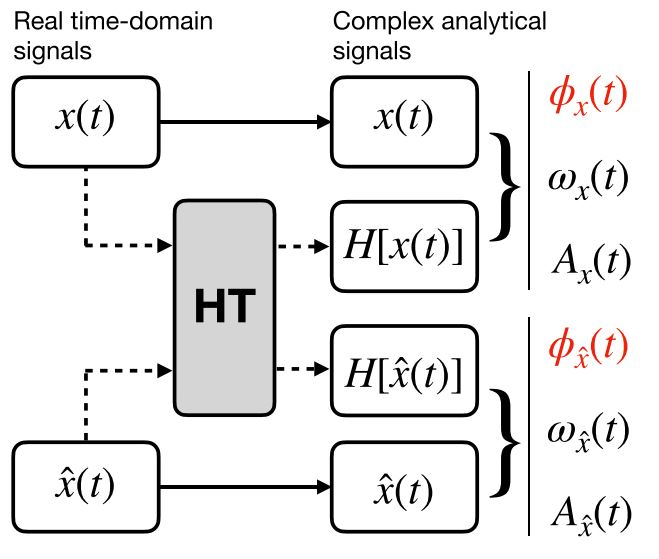Journal

# Estimating phase error using a Hilbert transform-based time-domain technique

By
Al-Ali A.A.
Elwakil A.S.
Maundy B.J.
Allagui A.
Elamien M.B.

Measuring phase noise in oscillators is crucial in communication systems, vibration analysis, and frequency synthesizers. Traditionally, this measurement is done in frequency domain by estimating the ratio of the power density at an offset frequency from the carrier to the power of the carrier signal. This approach is hardware intensive and dependent on the the offset frequency, for which there exists no standard. Here, we propose an alternative method to quantify phase noise but in the time domain in the form of a root-mean-square true phase angle deviation. This is done using a self-created reference signal and by applying the Hilbert transform to generate a complex analytic signal from the real time-domain data sequence. This enables the computation of instantaneous metrics such as phase noise. Simulations and experimental results are provided to validate the proposed technique. In addition, its relationship to the origin of phase noise is mathematically explained. © 2021 John Wiley & Sons, Ltd.